## Elliptic Hyperboloid

The elliptic hyperboloid is the generalization of the Hyperboloid to three distinct semimajor axes. The elliptic hyperboloid of one sheet is a Ruled Surface and has Cartesian equation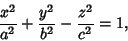(1)

and parametric equations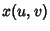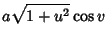(2)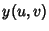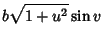(3)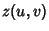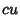(4)

for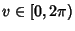, or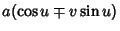(5)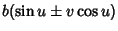(6)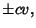(7)

or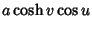(8)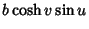(9)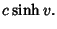(10)

The two-sheeted elliptic hyperboloid oriented along the z-Axis has Cartesian equation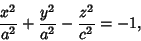(11)

and parametric equations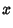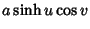(12)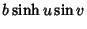(13)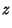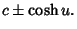(14)

The two-sheeted elliptic hyperboloid oriented along the x-Axis has Cartesian equation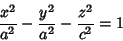(15)

and parametric equations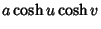(16)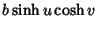(17)(18)